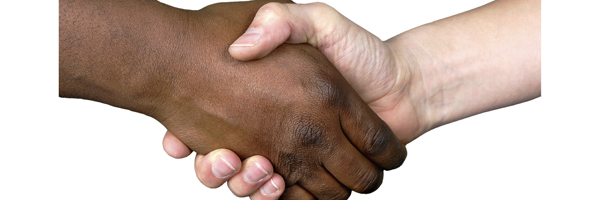# Shake to SolveSometimes real progress in maths comes when you find a way of looking at a problem in two different ways. Here is a great example of this.

Suppose you have $10$ people in a room and each person shakes hands with each other person once. How many handshakes do you get in total? The first person shakes hands with $9$ other people, the second shakes hands with the $8$ remaining people, the third shakes hands with $7$ remaining people, etc, giving a total of

$$9+8+7+...+2+1$$ handshakes.

But we can also look at this in another way: each person shakes hands with $9$ others and there are $10$ people, giving $10 \times 9 = 90$ handshakes. But this counts every handshake twice, so we need to divide by 2, giving a total of

$$\frac{10 \times 9}{2} = 45$$ handshakes.

Putting these two arguments together, we have just come up with a quick way of summing the first $9$ whole numbers. Rather than doing lots of adding, we only need to multiply $10$ by $9$ and then divide by $2$.

The same reasoning works more generally. If you there are $n+1$ people in the room, then the first argument shows that there are

$$n+(n-1)+(n-2)+...+2+1$$ handshakes, and the second one shows that there are
$$\frac{n \times (n+1)}{2}$$ handshakes.

This shows that

$$n+(n-1)+(n-2)+...+2+1 = \frac{n \times (n+1)}{2}.$$

In other words, our two ways of thinking have produced a formula for summing the first $n$ whole numbers and proved that the formula is correct.